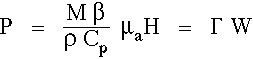# Thermoelastic expansion

The pressure generated in an object by thermoelastic expansion due to a very short laser pulse is described:M
= Bulk Modulus [Pa/strain], strain is dimensionless
= ρcs2,
cs
= sound velocity [m/s]
β
= Thermal expansivity [strain/degreeC], strain is dimensionless
ρ
= Density [kg/m3]
Cp
= Specific heat [(J/(kg degC)]
Γ
= Grueneisen coefficient [dimensionless]
μa
= Absorption coefficient [m-1]
H
W
= Energy depostion [J/m3]

The energy deposition W = μaH [J/m3].

The temperature rise ΔT = (energy deposition)/(rho Cp) [degree C].

The strain ε = βΔT [dimensionless].

The stress or pressure = Mε [J/m3] = [kg/(m s2] = [Pa].

The Grueneisen coefficient Γ = Mβ/(ρ Cp) [dimensionless].

The units of pressure (P) are (force)/(area). The units of energy deposition (W) are (energy)/(volume) = (force x distance)/(area x distance) in which the distance term cancels to equal (force)/(area). Hence the units of pressure and energy deposition are identical. The conversion factor for pressure is 1 J/m3 = 1 Pa = 10-5 [bar].